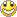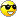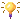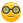Some new stuff
1. Arduino Park Control
2. Arduino USB2serial programming the bare bone
3. Arduino Projects Videos added
4. Arduino Xbee projects
5. Arduino dB Meter
6. Trying to get the IOIO going.
7. IOIO is going....bluetooth8. Attiny 2313 bluetooth bee RC car
9. Compass and temperature added
10. AVR programmer
11. USB2Serial and Ethernet POE
12. Arduino LPG Sensor
13. Program the Arduino Mini 05
14. Lipo Rider Pro in action
15. EZ Robot Builder kit arrived 10 Nov 2012
16. Arduino VA Meter!
17. 05 Nov 2012 Project Sentry Gun.
18. Attiny85 PIR Sensor 10 Dec 2012
19. 0-30VDC3A and 2-28VDC10A bench PSU's20. 19 Dec 2012 Arduino Voltmeter LCD
21. 29 Dec 2012 Arduino Tachometer&Speedometer LCD
22. 03 Jan 2013 Arduino AC Phase Control.
23. 18 Jan 2013 Arduino Distance Meter
24. 22 Jan 2013 Arduino Digital Clock and Date
25. 31 Jan 2013 Arduino Digital Clock and Date 8x2 LCD
26. Feb 2013 LCD and Backpack
27. Feb 2013 7 Segment Serial Clock Sparkfun. Distance Sensor with "newping" library
28. Feb 2013 Lelo Remote
29. 01/03/2013 Arduino Adafruit 7 Seg Digital Clock with RTC and backpack
30. Arduino Frequency Meter 26/03/2013
31. Another RC  Car controlled by Arduino 06/04/2013
32. My quadcopter project update 25 April 2013
33. My Aqua Quad Copter Flying 26 April 201334. Another monster RC truck;strong one! 11July201335. X-Frame Quad Copter 31 July 201336. Arduino GSM Sheild added.28/8/2013
37. 1.8inch TFT screen 13/09/201338. 04/11/2014 added PID Soldering Iron Control
39. Added bluetooth servo control 04/11/2014
40. ESP8266 first try.16/03/2015
41. Arduino Uno Quadcopter 19 May 2015
42. IOT WemosD1 Amp MeterHCM6352 Sparkfun Compass 8by2 LCD.docx

HCM6352 Sparkfun Compass 8by2 LCD

Arduino compass with Sparkfun HMC 6352 combined with 8x2 lcd.

I have added three lines to the coding to display the direction with leds if needed. See code at the bottom of the page.

Code:

#include <Wire.h>
#include <LiquidCrystal.h>
int HMC6352Address = 0x42;
// This is calculated in the setup() function
int slaveAddress;
int ledPin = 13;
boolean ledState = false;
byte headingData;
int i, headingValue;
LiquidCrystal lcd(8,9,4,5,6,7);

void setup()
{
// Shift the device's documented slave address (0x42) 1 bit right
// This compensates for how the TWI library only wants the
// 7 most significant bits (with the high bit padded with 0)
slaveAddress = HMC6352Address >> 1;   // This results in 0x21 as the address to pass to TWI
Serial.begin(9600);
pinMode(ledPin, OUTPUT);      // Set the LED pin as output
Wire.begin();
lcd.begin(8,2);
}
void loop()
{
// Flash the LED on pin 13 just to show that something is happening
// Also serves as an indication that we're not "stuck" waiting for TWI data
ledState = !ledState;
if (ledState) {
digitalWrite(ledPin,HIGH);
}
else
{
digitalWrite(ledPin,LOW);
}
// Send a "A" command to the HMC6352
// This requests the current heading data
Wire.beginTransmission(slaveAddress);
Wire.write("A");              // The "Get Data" command
Wire.endTransmission();
delay(10);                   // The HMC6352 needs at least a 70us (microsecond) delay
// after this command.  Using 10ms just makes it safe
// Read the 2 heading bytes, MSB first
// The resulting 16bit word is the compass heading in 10th's of a degree
// For example: a heading of 1345 would be 134.5 degrees
Wire.requestFrom(slaveAddress, 2);        // Request the 2 byte heading (MSB comes first)
i = 0;
while(Wire.available() && i < 2)
{
headingData[i] = Wire.read();
i++;
}
headingValue = headingData*256 + headingData;  // Put the MSB and LSB together
int gradi = int(headingValue / 10);
int fraction = int(headingValue % 10);
lcd.clear();
Serial.print("Current heading: ");
Serial.print(gradi); lcd.print(gradi);    // The whole number part of the heading
Serial.print("."); lcd.write('.');
Serial.print(fraction); lcd.print(fraction);    // The fractional part of the heading
Serial.print(" degrees Direction: ");
lcd.print((char)223);     // Output the degrees character
lcd.setCursor(0,1);
if ((gradi >= 337 && gradi <= 359) || (gradi >= 0 && gradi < 22))
{ Serial.println("N"); lcd.print("North");}
else if (gradi >= 292 && gradi <= 336)
{ Serial.println("NW"); lcd.print("N-West");}
else if (gradi >= 252 && gradi <= 291)
{ Serial.println("W"); lcd.print("West");}
else if (gradi >= 207 && gradi <= 251)
{ Serial.println("SW"); lcd.print("S-West");}
else if (gradi >= 157 && gradi <= 206)
{ Serial.println("S"); lcd.print("South");}
else if (gradi >= 112 && gradi <= 156)
{ Serial.println("SE"); lcd.print("S-East");}
else if (gradi >= 67 && gradi <= 111)
{ Serial.println("E"); lcd.print("East");}
else if (gradi >= 22 && gradi <= 66)
{ Serial.println("NE"); lcd.print("N-East");}
else
Serial.println("");

delay(500);The code with LED lines added:

//A4 = SDA
//A5 = SCL
#include<Wire.h>
#include <LiquidCrystal.h>
int HMC6352Address = 0x42;
// This is calculated in the setup() function
int slaveAddress;
int ledPin = 13;
int ledPin2 = 2;
int ledPin3 = 3;
int ledPin10 = 10;
boolean ledState = false;
byte headingData;
int i, headingValue;
LiquidCrystal lcd(8,9,4,5,6,7);

void setup()
{
// Shift the device's documented slave address (0x42) 1 bit right
// This compensates for how the TWI library only wants the
// 7 most significant bits (with the high bit padded with 0)
slaveAddress = HMC6352Address >> 1;   // This results in 0x21 as the address to pass to TWI
Serial.begin(9600);
pinMode(ledPin, OUTPUT);      // Set the LED pin as output
Wire.begin();
lcd.begin(8,2);
}
void loop()
{
// Flash the LED on pin 13 just to show that something is happening
// Also serves as an indication that we're not "stuck" waiting for TWI data
ledState = !ledState;
if (ledState) {
digitalWrite(ledPin,HIGH);
}
else
{
digitalWrite(ledPin,LOW);
}
// Send a "A" command to the HMC6352
// This requests the current heading data
Wire.beginTransmission(slaveAddress);
Wire.write("A");              // The "Get Data" command
Wire.endTransmission();
delay(10);                   // The HMC6352 needs at least a 70us (microsecond) delay
// after this command.  Using 10ms just makes it safe
// Read the 2 heading bytes, MSB first
// The resulting 16bit word is the compass heading in 10th's of a degree
// For example: a heading of 1345 would be 134.5 degrees
Wire.requestFrom(slaveAddress, 2);        // Request the 2 byte heading (MSB comes first)
i = 0;
while(Wire.available() && i < 2)
{
headingData[i] = Wire.read();
i++;
}
headingValue = headingData*256 + headingData;  // Put the MSB and LSB together
int gradi = int(headingValue / 10);
int fraction = int(headingValue % 10);
lcd.clear();
Serial.print("Current heading: ");
Serial.print(gradi); lcd.print(gradi);    // The whole number part of the heading
Serial.print("."); lcd.write('.');
Serial.print(fraction); lcd.print(fraction);    // The fractional part of the heading
Serial.print(" degrees Direction: "); lcd.print("deg");

lcd.setCursor(1,1);
if ((gradi >= 337 && gradi <= 359) || (gradi >= 0 && gradi < 22))
{ Serial.println("N"); lcd.print("North");
digitalWrite(ledPin2, HIGH);
delay(100);
digitalWrite(ledPin2, LOW);}
else if (gradi >= 292 && gradi <= 336)
{ Serial.println("NW"); lcd.print("N-West");
digitalWrite(ledPin3, HIGH);
delay(100);
digitalWrite(ledPin3, LOW);}
else if (gradi >= 252 && gradi <= 291)
{ Serial.println("W"); lcd.print("West");
digitalWrite(ledPin10, HIGH);
delay(100);
digitalWrite(ledPin10, LOW);}
else if (gradi >= 207 && gradi <= 251)
{ Serial.println("SW"); lcd.print("S-West");}
else if (gradi >= 157 && gradi <= 206)
{ Serial.println("S"); lcd.print("South");}
else if (gradi >= 112 && gradi <= 156)
{ Serial.println("SE"); lcd.print("S-East");}
else if (gradi >= 67 && gradi <= 111)
{ Serial.println("E"); lcd.print("East");}
else if (gradi >= 22 && gradi <= 66)
{ Serial.println("NE"); lcd.print("N-East");}
else
Serial.println("");
delay(500);Back Back to top